8th Grade Math Vocabulary Flashcards

8th Grade Math Vocabulary Flashcards
1/15 (missed) 0 0
Create Your Account To Continue Studying

As a member, you'll also get unlimited access to over 79,000 lessons in math, English, science, history, and more. Plus, get practice tests, quizzes, and personalized coaching to help you succeed.

Try it risk-free for 30 days. Cancel anytime
Hypotenuse
The longest side of a right angle, opposite of the 90-degree angle, is the hypotenuse.
Got it
Cube
Taking a number to the third power is the cube of that number. 4 cubed = 4 x 4 x 4 = 64
Got it
Counterclockwise
Rotating figures on a coordinate plane in the opposite direction as the hands on a clock are counterclockwise.
Got it
Clockwise
Rotating figures on a coordinate plane in the same direction as the hands on a clock are clockwise.
Got it
coordinate
A point (x,y) on a plane is a coordinate.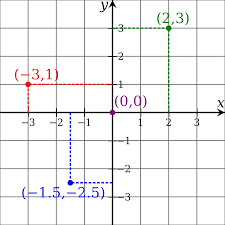Got it
Identify this type of angle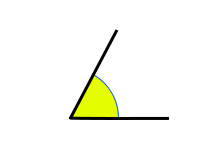An angle that is greater than 0 degrees and less than 90 degrees is an acute angle.
Got it
absolute value
The distance a number is from zero is the absolute value. The number is always positive. The absolute value of |30| and |-30| is 30.
Got it

Ready to move on?

or choose a specific lesson: See all lessons in this chapter
15 cards in set

Flashcard Content Overview

Math is not just about numbers. It is also made up of words and concepts. Just as you have learned math terms in the previous grade levels, there are words and concepts that will be used in the 8th grade. These flashcards will help you learn the words and their definitions. In some cases, you will be shown a graphic that will need to be identified. In other cases, you will need to know the definition. The terms in this flashcard set are just the basic concepts that will be used in the 8th grade, and beyond. You may also be introduced to additional words and concepts as you continue in this class. They will also be used as you continue in your future math classes.

Front
Back
absolute value
The distance a number is from zero is the absolute value. The number is always positive. The absolute value of |30| and |-30| is 30.
Identify this type of angleAn angle that is greater than 0 degrees and less than 90 degrees is an acute angle.
coordinate
A point (x,y) on a plane is a coordinate.Clockwise
Rotating figures on a coordinate plane in the same direction as the hands on a clock are clockwise.
Counterclockwise
Rotating figures on a coordinate plane in the opposite direction as the hands on a clock are counterclockwise.
Cube
Taking a number to the third power is the cube of that number. 4 cubed = 4 x 4 x 4 = 64
Hypotenuse
The longest side of a right angle, opposite of the 90-degree angle, is the hypotenuse.
Identify this type of angle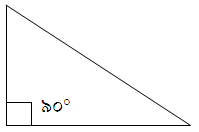An angle that is exactly 90-degrees is called a right angle.
Identify this type of angle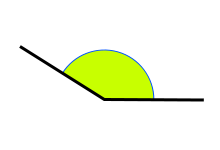An angle that is greater than 90 degrees but less than 180 degrees is an obtuse angle.
Identify the following lines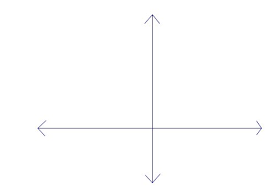Lines that cross each other while forming a right angle are perpendicular lines.
Identify the following lines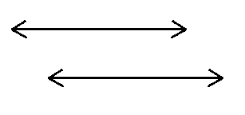Two lines that lie near each other and will never intersect are called parallel lines.
Rate
A comparison of the division between two different units of measure is called a rate.
Reciprocal
The multiplicative inverse of a number is its reciprocal. 1/x is the reciprocal of x/1 or x, and 4/5 is the reciprocal of 5/4.
variable
A letter that is used to represent an unknown number is called a variable. In the example, 2b +5, b is the variable.
non-parallel lines
Lines that will eventually intersect are non-parallel lines.

To unlock this flashcard set you must be a Study.com Member.